# US20160211760A1 - Transformer - Google Patents

## Info

Publication number
US20160211760A1
US20160211760A1 US14/912,568 US201414912568A US2016211760A1 US 20160211760 A1 US20160211760 A1 US 20160211760A1 US 201414912568 A US201414912568 A US 201414912568A US 2016211760 A1 US2016211760 A1 US 2016211760A1
Authority
US
United States
Prior art keywords
transformer
lines
circuit
terminal pair
line
Prior art date
Legal status (The legal status is an assumption and is not a legal conclusion. Google has not performed a legal analysis and makes no representation as to the accuracy of the status listed.)
Granted
Application number
US14/912,568
Other versions
US10320305B2 (en
Inventor
Kenichi Hirotsu
Nobuo Shiga
Hideaki Nakahata
Takashi Ohira
Current Assignee (The listed assignees may be inaccurate. Google has not performed a legal analysis and makes no representation or warranty as to the accuracy of the list.)
Toyohashi University of Technology NUC
Sumitomo Electric Industries Ltd
Original Assignee
Toyohashi University of Technology NUC
Sumitomo Electric Industries Ltd
Priority date (The priority date is an assumption and is not a legal conclusion. Google has not performed a legal analysis and makes no representation as to the accuracy of the date listed.)
Filing date
Publication date
Priority to JP2013178494A priority Critical patent/JP5695712B2/en
Priority to JP2013-178494 priority
Application filed by Toyohashi University of Technology NUC, Sumitomo Electric Industries Ltd filed Critical Toyohashi University of Technology NUC
Priority to PCT/JP2014/070967 priority patent/WO2015029744A1/en
Assigned to SUMITOMO ELECTRIC INDUSTRIES, LTD., NATIONAL UNIVERSITY CORPORATION TOYOHASHI UNIVERSITY OF TECHNOLOGY reassignment SUMITOMO ELECTRIC INDUSTRIES, LTD. ASSIGNMENT OF ASSIGNORS INTEREST (SEE DOCUMENT FOR DETAILS). Assignors: OHIRA, TAKASHI, YAMADA, KYOHEI, SHIGA, NOBUO, HIROTSU, KENICHI, NAKAHATA, HIDEAKI
Publication of US20160211760A1 publication Critical patent/US20160211760A1/en
Publication of US10320305B2 publication Critical patent/US10320305B2/en
Application granted granted Critical
Active legal-status Critical Current

• 238000010586 diagrams Methods 0.000 description 55
• 230000001131 transforming Effects 0.000 description 14
• 239000003990 capacitor Substances 0.000 description 12
• 230000003071 parasitic Effects 0.000 description 3
• 238000005549 size reduction Methods 0.000 description 3
• 229910002601 GaN Inorganic materials 0.000 description 2
• 280000024908 Next Generation companies 0.000 description 2
• 230000001939 inductive effects Effects 0.000 description 2
• 229910003465 moissanite Inorganic materials 0.000 description 2
• 229910010271 silicon carbide Inorganic materials 0.000 description 2
• 281000076521 Chubu Electric Power companies 0.000 description 1
• 230000005540 biological transmission Effects 0.000 description 1
• 230000001808 coupling Effects 0.000 description 1
• 238000010168 coupling process Methods 0.000 description 1
• 238000005859 coupling reactions Methods 0.000 description 1
• 230000000694 effects Effects 0.000 description 1
• 230000004048 modification Effects 0.000 description 1
• 238000006011 modification reactions Methods 0.000 description 1
• 239000003921 oils Substances 0.000 description 1
• 239000004065 semiconductors Substances 0.000 description 1

## Images

•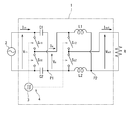•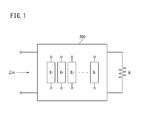•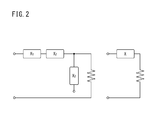•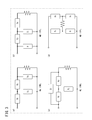•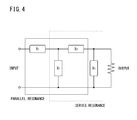•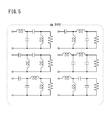•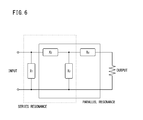•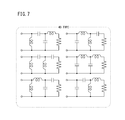••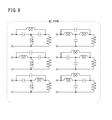•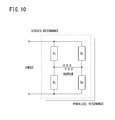•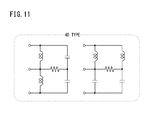••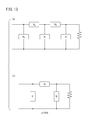•••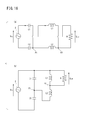•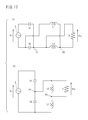••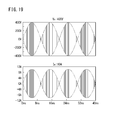•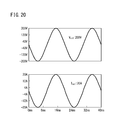•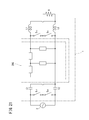•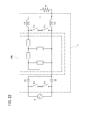•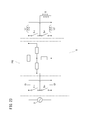•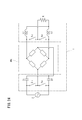## Classifications

• HELECTRICITY
• H02GENERATION; CONVERSION OR DISTRIBUTION OF ELECTRIC POWER
• H02MAPPARATUS FOR CONVERSION BETWEEN AC AND AC, BETWEEN AC AND DC, OR BETWEEN DC AND DC, AND FOR USE WITH MAINS OR SIMILAR POWER SUPPLY SYSTEMS; CONVERSION OF DC OR AC INPUT POWER INTO SURGE OUTPUT POWER; CONTROL OR REGULATION THEREOF
• H02M5/00Conversion of ac power input into ac power output, e.g. for change of voltage, for change of frequency, for change of number of phases
• H02M5/02Conversion of ac power input into ac power output, e.g. for change of voltage, for change of frequency, for change of number of phases without intermediate conversion into dc
• H02M5/04Conversion of ac power input into ac power output, e.g. for change of voltage, for change of frequency, for change of number of phases without intermediate conversion into dc by static converters
• H02M5/06Conversion of ac power input into ac power output, e.g. for change of voltage, for change of frequency, for change of number of phases without intermediate conversion into dc by static converters using impedances
• HELECTRICITY
• H02GENERATION; CONVERSION OR DISTRIBUTION OF ELECTRIC POWER
• H02MAPPARATUS FOR CONVERSION BETWEEN AC AND AC, BETWEEN AC AND DC, OR BETWEEN DC AND DC, AND FOR USE WITH MAINS OR SIMILAR POWER SUPPLY SYSTEMS; CONVERSION OF DC OR AC INPUT POWER INTO SURGE OUTPUT POWER; CONTROL OR REGULATION THEREOF
• H02M5/00Conversion of ac power input into ac power output, e.g. for change of voltage, for change of frequency, for change of number of phases
• H02M5/02Conversion of ac power input into ac power output, e.g. for change of voltage, for change of frequency, for change of number of phases without intermediate conversion into dc
• H02M5/04Conversion of ac power input into ac power output, e.g. for change of voltage, for change of frequency, for change of number of phases without intermediate conversion into dc by static converters
• H02M5/22Conversion of ac power input into ac power output, e.g. for change of voltage, for change of frequency, for change of number of phases without intermediate conversion into dc by static converters using discharge tubes with control electrode or semiconductor devices with control electrode
• H02M5/275Conversion of ac power input into ac power output, e.g. for change of voltage, for change of frequency, for change of number of phases without intermediate conversion into dc by static converters using discharge tubes with control electrode or semiconductor devices with control electrode using devices of a triode or transistor type requiring continuous application of a control signal
• H02M5/293Conversion of ac power input into ac power output, e.g. for change of voltage, for change of frequency, for change of number of phases without intermediate conversion into dc by static converters using discharge tubes with control electrode or semiconductor devices with control electrode using devices of a triode or transistor type requiring continuous application of a control signal using semiconductor devices only
• HELECTRICITY
• H03BASIC ELECTRONIC CIRCUITRY
• H03HIMPEDANCE NETWORKS, e.g. RESONANT CIRCUITS; RESONATORS
• H03H7/00Multiple-port networks comprising only passive electrical elements as network components
• H03H7/38Impedance-matching networks

## Abstract

A transformer is provided between a power supply, and a load with a resistance value R, and includes a two-terminal pair circuit composed of n-number of reactance elements that are mutually connected, where n is a natural number equal to or greater than 4. With respect to any value of the resistance value R of the load, an input impedance Zin of the two-terminal pair circuit has a real number component of k·R, where k is a constant, and an imaginary number component of 0. Such a transformer has a small size and a light weight, and does not need a coil, an iron core, and the like as used in a conventional transformer.

## Description

TECHNICAL FIELD
• The present invention relates to a transformer.
• BACKGROUND ART
• In a commercial AC transmission and distribution system, a transformer is used. Just near a consumer's house, a pole transformer is used which transforms, for example, 6600 V (50 Hz or 60 Hz) to 200 V (see NON PATENT LITERATURE 1). Such a pole transformer has a thick coil as a conductive wire wound around an iron core, and therefore has a considerable weight. For example, a pole transformer with a diameter of 40 cm and a height of 80 cm has a weight of about 200 kg, including an insulating oil and a case.
• On the other hand, for realizing a smart grid which is a next-generation power system, studies of an SST (Solid-State Transformer) are being conducted. For the SST, a high-frequency transformer is used (for example, see NON PATENT LITERATURE 2).
• CITATION LIST Non Patent Literature
• NON PATENT LITERATURE 1: Homepage of Chubu Electric Power Co., Inc., [Pole Transformer], [online], [searched on Jul. 19, 2013], Internet <URL:http://www.chuden.co.jp/e-museum/guide/3floor/exhibit_c23.html>
• NON PATENT LITERATURE 2: Falcones, S.: et al., Power and Energy Society General Meeting, 2010 IEEE, pp. 1-8, Minneapolis, July 2010
• SUMMARY OF INVENTION Technical Problem
• A conventional pole transformer is heavy, and therefore is not easy to handle. In addition, an attachment space that is large enough to contain the outer dimension of the transformer is needed on the pole.
• On the other hand, a high-frequency transformer cannot avoid an influence of a parasitic capacitance, and has a difficulty in designing.
• Considering such conventional problems, an object of the present invention is to provide an innovative next-generation transformer with a small size and a light weight, which does not need a coil, an iron core, and the like for magnetic coupling, electromagnetic induction, or mutual inductance as used in a conventional transformer.
• Solution to Problem
• The present invention is a transformer provided between a power supply, and a load with a resistance value R, the transformer including a two-terminal pair circuit composed of n-number of reactance elements that are mutually connected, where n is a natural number equal to or greater than 4, wherein, with respect to any value of the resistance value R of the load, an input impedance Zin of the two-terminal pair circuit has a real number component of k·R, where k is a constant, and an imaginary number component of 0.
• It is noted that a reactance element is an inductor having an inductive reactance or a capacitor having a capacitive reactance.
• Using the transformer of the present invention as a power transformer makes it unnecessary to use a conventional transformer including a coil, an iron core, and the like. Therefore, it becomes possible to realize drastic size reduction and weight reduction of a transformer, and thereby realize cost reduction.
• BRIEF DESCRIPTION OF DRAWINGS
• FIG. 1 is a diagram showing a concept of a transformer using a two-terminal pair circuit (four-terminal circuit).
• FIG. 2 is a diagram showing a concept of the element number of elements composing a circuit.
• FIG. 3 is a diagram showing four patterns of circuit configurations that can be made by using the minimum element number 4.
• FIG. 4 is a diagram showing a circuit configuration of 4A type.
• FIG. 5 is a diagram showing six patterns as actual examples of the circuit configuration of 4A type.
• FIG. 6 is a diagram showing a circuit configuration of 4B type.
• FIG. 7 is a diagram showing six patterns as actual examples of the circuit configuration of 4B type.
• FIG. 8 is a diagram showing a circuit configuration of 4C type.
• FIG. 9 is a diagram showing six patterns as actual examples of the circuit configuration of 4C type.
• FIG. 10 is a diagram showing a circuit configuration of 4D type.
• FIG. 11 is a diagram showing two patterns as actual examples of the circuit configuration of 4D type.
• FIG. 12 is a circuit diagram in which (a) shows a first example of circuit configuration with n=5, and (b) shows a T-shaped circuit.
• FIG. 13 is a circuit diagram in which (a) shows a second example of circuit configuration with n=5, and (b) shows a i-shaped circuit.
• FIG. 14 is a circuit diagram in which (a) shows a first example of circuit configuration with n=6, and (b) shows a second example of circuit configuration with n=6.
• FIG. 15 is a circuit diagram showing a transformer having another configuration.
• FIG. 16 is a circuit diagram in which (a) shows a substantial connection state when, of four switches in FIG. 15, two switches on the upper side are ON and two switches on the lower side are OFF, and (b) shows, in a step-like shape, the same circuit diagram as in (a).
• FIG. 17 is a circuit diagram in which (a) shows a substantial connection state when, of the four switches in FIG. 15, the two switches on the lower side are ON and the two switches on the upper side are OFF, and (b) shows, in a step-like shape, the same circuit diagram as in (a).
• FIG. 18 is waveform diagrams respectively showing input voltage and input current to the transformer in FIG. 15.
• FIG. 19 is waveform diagrams respectively showing voltage and current at an intermediate stage of transformation in the transformer in FIG. 15.
• FIG. 20 is waveform diagrams respectively showing output voltage and output current from the transformer in FIG. 15.
• FIG. 21 is a circuit diagram showing a combination of the transformer shown in FIG. 15 and the transformer having the circuit configuration of 4A type.
• FIG. 22 is a circuit diagram showing a combination of the transformer shown in FIG. 15 and the transformer having the circuit configuration of 4B type.
• FIG. 23 is a circuit diagram showing a combination of the transformer shown in FIG. 15 and the transformer having the circuit configuration of 4C type.
• FIG. 24 is a circuit diagram showing a combination of the transformer shown in FIG. 15 and the transformer having the circuit configuration of 4D type.
• DESCRIPTION OF EMBODIMENTS Summary of Embodiments
• Summary of embodiments of the present invention includes at least the following.
• (1) A transformer is provided between a power supply, and a load with a resistance value R, and includes a two-terminal pair circuit composed of n-number of reactance elements that are mutually connected, where n is a natural number equal to or greater than 4. With respect to any value of the resistance value R of the load, an input impedance Zin of the two-terminal pair circuit has a real number component of k·R, where k is a constant, and an imaginary number component of 0.
• The transformer as described above can obtain output voltage proportional to input voltage regardless of the resistance value R of the load. That is, the transformer that transforms input voltage to output voltage with a constant voltage transformation ratio (1/k)1/2 can be obtained. Using the above transformer as transformers makes it unnecessary to use a conventional commercial-frequency transformer or high-frequency transformer. Therefore, drastic size reduction and weight reduction of a transformer can be realized, and as a result, cost reduction can be realized. Further, problems of parasitic capacitance and occurrence of magnetic field leakage, which arise in a high-frequency transformer, are also solved, and thus a transformer with low loss can be realized.
• (2) In the transformer of (1), preferably, in the case where reactances of four reactance elements are X1, X2, X3, and X4, the two-terminal pair circuit is composed of, starting from an input side, X1 present on one line of the two-terminal pair circuit, X2 present between two lines thereof, X3 present on the one line, and X4 present between the two lines, and the following condition is satisfied.
• In this case, the input impedance Zin is represented as Zin=(X2 2/X4 2)·R, and is proportional to the resistance value R of the load, and thus output voltage proportional to input voltage is obtained.
• It is noted that the symbol “” denotes a logical conjunction or AND (hereafter, the same applies).
• (3) In the transformer of (1), preferably, in the case where reactances of four reactance elements are X1, X2, X3, and X4, the two-terminal pair circuit is composed of, starting from an input side, X1 present between two lines of the two-terminal pair circuit, X2 present on one line thereof, X3 present between the two lines, and X4 present on the one line, and the following condition is satisfied.
• In this case, the input impedance Zin is represented as Zin=(X1 2/X3 2)·R, and is proportional to the resistance value R of the load, and thus output voltage proportional to input voltage is obtained.
• (4) In the transformer of (1), preferably, in the case where reactances of four reactance elements are X1, X2, X3, and X4, the two-terminal pair circuit is composed of: starting from an input side, a T-shaped circuit formed by X1 present on one line of the two-terminal pair circuit, X2 present between two lines thereof, and X3 present on the one line; and X4 present in parallel with a series unit of X1 and X3, and the following condition is satisfied.
• In this case, the input impedance Zin is represented as Zin=(X1 2/X3 2)·R, and is proportional to the resistance value R of the load, and thus output voltage proportional to input voltage is obtained.
• (5) In the transformer of (1), preferably, in the case where reactances of four reactance elements are X1, X2, X3, and X4, the two-terminal pair circuit is composed of, starting from an input side, a first series unit of X1 and X2 present between two lines of the two-terminal pair circuit, and a second series unit of X3 and X4 present between the two lines, such that a mutual connection point in the first series unit and a mutual connection point in the second series unit are output terminals, and the following condition is satisfied.
• In this case, the input impedance Zin is represented as Zin={(X1+X2)2/(X1−X2)2}·R, and is proportional to the resistance value R of the load, and thus output voltage proportional to input voltage is obtained.
• (6) In the transformer of (1), preferably, in the case where reactances of five reactance elements are XA, XB, XC, XD, and XE, the two-terminal pair circuit is composed of, starting from an input side, XA present on one line of the two-terminal pair circuit, XB present between two lines thereof, XC present on the one line, XD present between the two lines, and XE present on the one line, and the following relationship is satisfied.
• In this case, the input impedance Zin is represented as Zin=(XA 2/XE 2)·R, and is proportional to the resistance value R of the load, and thus output voltage proportional to input voltage is obtained.
• (7) In the transformer of (1), preferably, in the case where reactances of five reactance elements are XA, XB, XC, XD, and XE, the two-terminal pair circuit is composed of, starting from an input side, XA present between two lines of the two-terminal pair circuit, XB present on one line thereof, XC present between the two lines, XD present on the one line, and XE present between the two lines, and the following relationship is satisfied.
• In this case, the input impedance Zin is represented as Zin=(XA 2/XE 2)·R, and is proportional to the resistance value R of the load, and thus output voltage proportional to input voltage is obtained.
• (8) In the transformer of (1), preferably, in the case where reactances of six reactance elements are XA, XB, XC, XD, XE, and XF, the two-terminal pair circuit is composed of, starting from an input side, XA present on one line of the two-terminal pair circuit, XB present between two lines thereof, XC present on the one line, XD present between the two lines, XE present on the one line, and XF present between the two lines, and the following relationship is satisfied.
• In this case, the input impedance Zin is represented as Zin=(XA 2/XF 2)·R, and is proportional to the resistance value R of the load, and thus output voltage proportional to input voltage is obtained.
• (9) In the transformer of (1), preferably, in the case where reactances of six reactance elements are XA, XB, XC, XD, XE, and XF, the two-terminal pair circuit is composed of, starting from an input side, XA present between two lines of the two-terminal pair circuit, XB present on one line thereof, XC present between the two lines, XD present on the one line, XE present between the two lines, and XF present on the one line, and the following relationship is satisfied.
• In this case, the input impedance Zin is represented as Zin=(XA 2/XF 2)·R, and is proportional to the resistance value R of the load, and thus output voltage proportional to input voltage is obtained.
• (10) A transformer may include: a circuit configured to perform switching; and the transformer of any one of (1) to (9), which is interposed in the circuit.
• In this case, it is possible to utilize a transformer having a lumped constant circuit, using an environment in which switching is performed.
• (11) In the transformer of any one of (1) to (9), a capacitance of a cable and an inductance of a cable may be used as the reactance elements.
• In this case, the cable can easily ensure voltage withstanding performance and the cost thereof is low.
• (12) In the transformer of (10), preferably, a frequency of the switching is at least 1 MHz.
• In this case, it is possible to utilize a transformer having a lumped constant circuit, using an environment in which switching is performed at a high frequency.
• Details of Embodiments
• <Transformer Using Lumped Constant Circuit>
• Next, the details of a transformer using a lumped constant circuit according to embodiments of the present invention will be described.
• <<Outline>>
• FIG. 1 is a diagram showing a concept of a transformer 200 using a two-terminal pair circuit (four-terminal circuit). For functioning as a transformer, an input impedance Zin and a load R (resistance value R) need to satisfy the following relationship.

• Z in =k·R (k is a constant)
• Thus, the input impedance Zin linearly varies with respect to load variation, and the voltage transformation ratio is constant. In addition, the input impedance Zin does not have a reactance component. That is, the input impedance Zin needs to have a real number component of k·R and an imaginary number component of 0. The transformer 200 having such an input impedance Zin is referred to as an LILT (Load-Invariant Linear Transformer).
• The transformer 200 as described above can obtain output voltage proportional to input voltage regardless of the resistance value R of the load. That is, the transformer 200 that transforms input voltage to output voltage with a constant voltage transformation ratio (1/k)1/2 can be obtained. Using such a transformer 200 as transformers makes it unnecessary to use a conventional commercial-frequency transformer or high-frequency transformer. Therefore, drastic size reduction and weight reduction of a transformer can be realized, and as a result, cost reduction can be realized. Further, problems of parasitic capacitance and occurrence of magnetic field leakage, which arise in a high-frequency transformer, are also solved, and thus a transformer with low loss can be realized.
• Although an infinite number of circuit configurations as an LILT are conceivable, it is desirable that an element number n of reactance elements is small. The present inventors have performed full search while changing the value of n to 1, 2, 3, 4, . . . , starting from 1, and as a result, have found that the minimum element number n is 4.
• FIG. 2 is a diagram showing an example of a concept of the element number n of elements composing a circuit. In FIG. 2, the left circuit apparently includes three elements X1, X2, and X3. However, X1 and X2 which are topologically equivalent to each other are counted as one element, and X3 which has no significance in terms of electric circuit is not counted. Therefore, the left circuit configuration is the same as the right circuit configuration, and the element number n is 1.
• FIG. 3 is a diagram showing four patterns of circuit configurations that can be made by using the minimum element number 4, in which circuit configurations of (a), (b), (c), and (d) are referred to as “4A type”, “4B type”, “4C type”, and “4D type”, respectively.
• First Embodiment 4A Type
• FIG. 4 is a diagram showing the circuit configuration of 4A type. To describe in words, for example, in the case where reactances of the four reactance elements are X1, X2, X3, and X4, a two-terminal pair circuit is composed of, starting from the input side, X1 present on one line of the two-terminal pair circuit, X2 present between two lines thereof, X3 present on the one line, and X4 present between the two lines. In this case, the input impedance Zin is represented as the upper part of the following expression. In addition, in the case of setting a condition for causing the imaginary number component to be 0 by parallel resonance and series resonance, the input impedance Zin is represented as the lower part.
• In the following expression, “j” denotes an imaginary number (−1)1/2.
• $Z in = R  { X 1  ( X 2 + X 3 + X 4 ) + X 2  ( X 3 + X 4 ) + jX 4  ( X 1  X 2 + X 2  X 3 + X 3  X 1 ) X 4  ( X 2 + X 3 ) - jR  ( X 2 + X 3 + X 4 ) = X 2 2 X 4 2  R , ( 1 X 1 + 1 X 2 + 1 X 3 = 0 ⋀ X 2 + X 3 + X 4 = 0 )$
• That is, when the parameter condition satisfies (1/X1)+(1/X2)+(1/X3)=0X2+X3+X4=0, in other words, when (1/X1)+(1/X2)+(1/X3)=0 and X2+X3+X4=0 are satisfied, Zin=(X2 2/X4 2)·R is satisfied, and thus output voltage proportional to input voltage is obtained. It is noted that, in order to exert this function, the input voltage needs to be AC.
• FIG. 5 is a diagram showing six patterns as actual examples of the circuit configuration of 4A type.
• Second Embodiment 4B Type
• FIG. 6 is a diagram showing the circuit configuration of 4B type. To describe in words, for example, in the case where reactances of the four reactance elements are X1, X2, X3, and X4, a two-terminal pair circuit is composed of, starting from the input side, X1 present between two lines of the two-terminal pair circuit, X2 present on one line thereof, X3 present between the two lines, and X4 present on the one line. In this case, the input impedance Zin is represented as the upper part of the following expression. In addition, in the case of setting a condition for causing the imaginary number component to be 0 by parallel resonance and series resonance, the input impedance Zin is represented as the lower part.
• $Z in = RX 3  ( X 2 + X 3 ) + jX 1  ( X 2  X 3 + X 3  X 4 + X 4  X 2 ) X 3  ( X 1 + X 2 ) + X 4  ( X 1 + X 2 + X 3 ) - jR  ( X 1 + X 2 + X 3 ) = X 1 2 X 3 2  R , ( X 3 + X 2 + X 3 = 0 ⋀ 1 X 2 + 1 X 3 + 1 X 4 = 0 )$
• That is, when the parameter condition satisfies X1+X2+X3=0(1/X2)+(1/X3)+(1/X4)=0, Zin=(X1 2/X3 2)·R is satisfied, and thus output voltage proportional to input voltage is obtained. It is noted that, in order to exert this function, the input voltage needs to be AC.
• FIG. 7 is a diagram showing six patterns as actual examples of the circuit configuration of 4B type.
• Third Embodiment 4C type
• FIG. 8 is a diagram showing the circuit configuration of 4C type. To describe in words, for example, in the case where reactances of the four reactance elements are X1, X2, X3, and X4, a two-terminal pair circuit is composed of: starting from the input side, a T-shaped circuit formed by X1 present on one line of the two-terminal pair circuit, X2 present between two lines thereof, and X3 present on the one line; and X4 present in parallel with a series unit of Xi and X3. In this case, the input impedance Zin is represented as the upper part of the following expression. In addition, in the case of setting a condition for causing the imaginary number component to be 0 by parallel resonance and series resonance, the input impedance Zin is represented as the lower part.
• $Z in = - X 4  ( X 1  X 2 + X 2  X 3 + X 3  X 1 ) + jR  { X 1  ( X 3 + X 4 ) + X 2  ( X 1 + X 3 + X 4 ) } R  ( X 1 + X 3 + X 4 ) + j  { X 2  ( X 1 + X 3 + X 4 ) + X 3  ( X 1 + X 4 ) } = X 1 2 X 3 2  R , ( X 1 + X 3 + X 4 = 0 ⋀ 1 X 1 + 1 X 2 + 1 X 3 = 0 )$
• That is, when the parameter condition satisfies X1+X3+X4=0(1/X1)+(1/X2)+(1/X3)=0, Zin=(X1 2/X3 2)·R is satisfied, and thus output voltage proportional to input voltage is obtained. It is noted that, in order to exert this function, the input voltage needs to be AC.
• FIG. 9 is a diagram showing six patterns as actual examples of the circuit configuration of 4C type.
• Fourth Embodiment: 4D Type
• FIG. 10 is a diagram showing the circuit configuration of 4D type. To describe in words, for example, in the case where reactances of the four reactance elements are X1, X2, X3, and X4, a two-terminal pair circuit is composed of, starting from the input side, a first series unit of X1 and X2 present between two lines of the two-terminal pair circuit, and a second series unit of X3 and X4 present between the two lines, such that a mutual connection point in the first series unit and a mutual connection point in the second series unit are output terminals. In this case, the input impedance Zin is represented as the upper part of the following expression. In addition, in the case of setting a condition for causing the imaginary number component to be 0 by parallel resonance and series resonance, the input impedance Zin is represented as the lower part.
• $Z in =  - X 1  X 2  ( X 3 + X 4 ) - X 3  X 4  ( X 1 + X 2 ) + jR  ( X 1 + X 2 )  ( X 3 + X 4 ) R  ( X 1 + X 2 + X 3 + X 4 ) + j  ( X 1 + X 3 )  ( X 2 + X 4 ) } =  ( X 1 + X 2 ) 2 ( X 1 - X 2 ) 2  R , ( X 1 + X 2 + X 3 + X 4 =  0 ⋀ 1 X 1 + 1 X 2 + 1 X 3 + 1 X 4 = 0 )$
• That is, when the parameter condition satisfies X1+X2+X3+X4=0(1/X1)+(1/X2)+(1/X3)+(1/X4)=0, Zin={(X1+X2)2/(X1−X2)2}·R is satisfied, and thus output voltage proportional to input voltage is obtained. It is noted that, in order to exert this function, the input voltage needs to be AC.
• FIG. 11 is a diagram showing two patterns as actual examples of the circuit configuration of 4D type.
• Fifth Embodiment n=5 (Application of T Type)
• Next, the circuit configuration with the element number n=5 will be described. Although the element number increases by one from the case of n=4, this circuit configuration is practical.
• In FIG. 12, (a) is a diagram showing a first example of circuit configuration with n=5. To describe in words, in the case where reactances of the five reactance elements are XA, XB, XC, XD, and XE, a two-terminal pair circuit is composed of, starting from the input side, XA present on one line of the two-terminal pair circuit, XB present between two lines thereof, XC present on the one line, XD present between the two lines, and XE present on the one line.
• On the other hand, in FIG. 12, (b) shows a T-shaped circuit. In this T-shaped circuit, the input impedance Zin is represented as the upper part of the following expression, and in order to cause the imaginary number component to be 0, a parameter condition shown as the lower part needs to be satisfied.
• $Z in = - ( X 1  X 2 + X 2  X 3 + X 3  X 1 ) + jR  ( X 1 + X 2 ) R + j  ( X 2 + X 3 ) = X 1 2 R , ( X 1 = X 3 = - X 2 )$
• In the case of T-shaped circuit, since R is present in the denominator, an LILT is not obtained. However, if the T-shaped circuit is configured in a two-stage form, Zin=k·R is satisfied, and thus output voltage proportional to input voltage is obtained. Considering this, in the case where reactances of the five reactance elements in the circuit shown in (a) of FIG. 12 are XA, XB, XC, XD, and XE, the two-terminal pair circuit composed of, starting from the input side, XA present on one line of the two-terminal pair circuit, XB present between two lines thereof, XC present on the one line, XD present between the two lines, and XE present on the one line, is set to satisfy the following relationship.
• In this case, the input impedance Zin is represented as Zin =(XA 2/XE 2)·R, and is proportional to the resistance value R of the load, and thus output voltage proportional to input voltage is obtained. It is noted that, in order to exert this function, the input voltage needs to be AC.
• Sixth Embodiment n=5 (Application of π Type)
• In FIG. 13, (a) is a diagram showing a second example of circuit configuration with n=5. To describe in words, in the case where reactances of the five reactance elements are XA, XB, XC, XD, and XE, a two-terminal pair circuit is composed of, starting from the input side, XA present between two lines of the two-terminal pair circuit, XB present on one line thereof, XC present between the two lines, XD present on the one line, and XE present between the two lines.
• On the other hand, in FIG. 13, (b) shows a π-shaped circuit. In this n-shaped circuit, the input impedance Zin is represented as the upper part of the following expression, and in order to cause the imaginary number component to be 0, a parameter condition shown as the lower part needs to be satisfied.
• $Z in = RX 1  ( X 2 + X 3 ) + jX 1  X 2  X 3 X 3  ( X 1 + X 2 ) - jR  ( X 1 + X 2 + X 3 ) = X 1 2 R , ( X 1 = X 3 = - X 2 )$
• In the case of π-shaped circuit, since R is present in the denominator, an LILT is not obtained. However, if the π-shaped circuit is configured in a two-stage form, Zin=k·R is satisfied, and thus output voltage proportional to input voltage is obtained. Considering this, in the case where reactances of the five reactance elements in the circuit shown in (a) of FIG. 13 are XA, XB, XC, XD, and XE, the two-terminal pair circuit composed of, starting from the input side, XA present between two lines of the two-terminal pair circuit, XB present on one line thereof, XC present between the two lines, XD present on the one line, and XE present between the two lines, is set to satisfy the following relationship.
• In this case, the input impedance Zin is represented as Zin=(XA 2/XE 2)·R, and is proportional to the resistance value R of the load, and thus output voltage proportional to input voltage is obtained. It is noted that, in order to exert this function, the input voltage needs to be AC.
• Seventh Embodiment n=6 (T at Preceding Stage and π at Subsequent Stage)
• Next, the circuit configuration with the element number n=6 will be described. Although the element number increases by two from the case of n=4, this circuit configuration is practical.
• In FIG. 14, (a) is a diagram showing a first example of circuit configuration with n=6. To describe in words, in the case where reactances of the six reactance elements are XA, XB, XC, XD, XE, and XF, a two-terminal pair circuit is composed of, starting from the input side, XA present on one line of the two-terminal pair circuit, XB present between two lines thereof, XC present on the one line, XD present between the two lines, XE present on the one line, and XF present between the two lines.
• As described above, in either case of T-shaped circuit or π-shaped circuit, since R is present in the denominator, an LILT is not obtained. However, if the T-shaped circuit and the π-shaped circuit are combined, Zin=k·R is satisfied, and thus output voltage proportional to input voltage is obtained. Considering this, in the case where reactances of the six reactance elements in the circuit shown in (a) of FIG. 14 are XA, XB, XC, XD, XE, and XF, the two-terminal pair circuit composed of, starting from the input side, XA present on one line of the two-terminal pair circuit, XB present between two lines thereof, XC present on the one line, XD present between the two lines, XE present on the one line, and XF present between the two lines, is set to satisfy the following relationship.
• In this case, the input impedance Zin is represented as Zin=(XA 2/XE 2)·R, and is proportional to the resistance value R of the load, and thus output voltage proportional to input voltage is obtained. It is noted that, in order to exert this function, the input voltage needs to be AC.
• Eighth Embodiment n=6 (π at Preceding Stage and T at Subsequent Stage)
• In FIG. 14, (b) is a diagram showing a second example of circuit configuration with n=6. To describe in words, in the case where reactances of the six reactance elements are XA, XB, XC, XD, XE, and XF, a two-terminal pair circuit is composed of, starting from the input side, XA present between two lines of the two-terminal pair circuit, XB present on one line thereof, XC present between the two lines, XD present on the one line, XE present between the two lines, and XF present on the one line.
• As described above, in either case of π-shaped circuit or T-shaped circuit, since R is present in the denominator, an LILT is not obtained. However, if the π-shaped circuit and the T-shaped circuit are combined, Zin=k·R is satisfied, and thus output voltage proportional to input voltage is obtained. Considering this, in the case where reactances of the six reactance elements in the circuit shown in (b) of FIG. 14 are XA, XB, XC, XD, XE, and XF, the two-terminal pair circuit composed of, starting from the input side, XA present between two lines of the two-terminal pair circuit, XB present on one line thereof, XC present between the two lines, XD present on the one line, XE present between the two lines, and XF present on the one line, is set to satisfy the following relationship.
• In this case, the input impedance Zin is represented as Zin=(XA 2/XE 2)·R, and is proportional to the resistance value R of the load, and thus output voltage proportional to input voltage is obtained. It is noted that, in order to exert this function, the input voltage needs to be AC.
• The transformer 200 using a lumped constant circuit as described above may be used in combination with a transformer having another configuration. The following are examples of such a transformer having another configuration.
• <Transformer Using Switching by Reactance Element>
• FIG. 15 is a circuit diagram showing an example of such a transformer 1. In FIG. 15, the transformer 1 is provided between an AC power supply 2 and a load R. The transformer 1 includes a pair of capacitors C1 and C2, a pair of inductors L1 and L2, four switches Sr1, Sr2, Sb1, and Sb2, and a switching control section 3 which performs ON/OFF control of the switches Sr1, Sr2, Sb1, and Sb2. A switching frequency of the switching control section 3 is, for example, about 1 MHz. The switching frequency is preferably equal to or higher than 1 MHz, but may be lower than 1 MHz.
• The switches Sr1, Sr2, Sb1, and Sb2 and the switching control section 3 form a switch device 4 which switches the state of circuit connection of the transformer 1. The switches Sr1 and Sr2 operate in synchronization with each other, and the switches Sb1 and Sb2 operate in synchronization with each other. The pair of switches Sr1 and Sr2 and the pair of switches Sb1 and Sb2 operate so as to be alternately turned on exclusively from each other. The switches Sr1, Sr2, Sb1, and Sb2 are semiconductor switching elements formed by an SiC element or a GaN element, for example. An SiC element or a GaN element allows faster switching than an Si element, for example. In addition, sufficient withstand voltage (which can be even 6 kV per element, for example) can be obtained without connecting multiple stages of such elements.
• In FIG. 15, the pair of capacitors C1 and C2 are connected in series to each other via a connection point P1. The AC power supply 2 is connected between both ends of this series unit. The input voltage Vin is applied to the series unit of the pair of capacitors C1 and C2, so that input current Iin flows.
• The pair of inductors L1 and L2 are connected in series to each other via a connection point P2. Between both ends of this series unit, input voltage Vm is applied via the capacitors C1 and C2, so that input current Im flows. When one of the switches Sr1 and Sb2 is ON, current flows in the load R. Here, voltage applied to the load R is Vout, and output current flowing from the transformer 1 to the load R is Iout.
• In FIG. 16, (a) is a circuit diagram showing a substantial connection state when, of the four switches Sr1, Sr2, Sb1, and Sb2 in FIG. 15, the two switches Sr1 and Sr2 on the upper side are ON and the two switches Sb1 and Sb2 on the lower side are OFF. In the drawing, the switch device 4 in FIG. 15 is not shown. In FIG. 16, (b) is a circuit diagram showing, in a step-like shape, the same circuit diagram as in (a).
• On the other hand, in FIG. 17, (a) is a circuit diagram showing a substantial connection state when, of the four switches Sr1, Sr2, Sb1, and Sb2 in FIG. 15, the two switches Sb1 and Sb2 on the lower side are ON and the two switches Sr1 and Sr2 on the upper side are OFF. In FIG. 17, (b) is a circuit diagram showing, in a step-like shape, the same circuit diagram as in (a).
• While the states in FIG. 16 and FIG. 17 are alternately repeated, voltage obtained via the connection point P1 of the series unit of the capacitors C1 and C2 becomes voltage obtained via the connection point P2 of the series unit of the inductors L1 and L2. That is, transformation is performed from the capacitor side at the preceding stage to the inductor side at the subsequent stage. Here, it is estimated that the input voltage becomes about 1/4 to be outputted. This has been confirmed by experiments by the inventors.
• FIG. 18 is waveform diagrams, the upper one showing the input voltage to the transformer 1, and the lower one showing the input current to the transformer 1.
• FIG. 19 is waveform diagrams respectively showing the voltage Vm and the current Im at an intermediate stage of transformation. Each waveform is actually formed by a pulse train based on switching, and as a whole, represented by the waveform as shown in FIG. 19.
• FIG. 20 is waveform diagrams, the upper one showing the output voltage from the transformer 1, and the lower one showing the output current from the transformer 1. As is obvious from comparison between FIG. 18 and FIG. 20, the voltage is transformed to 1/4, and along with this, the current quadruples.
• Combination Examples
• FIG. 21 is a circuit diagram showing a combination of the transformer 1 shown in FIG. 15 and the transformer 200 having the circuit configuration of 4A type as described above. In FIG. 21, the transformer 200 is interposed between the capacitor stage and the inductor stage in the transformer 1 shown in FIG. 15. By thus combining transformation functions of the two kinds of transformers 1 and 200, it becomes possible to design the voltage transformation ratio in a wide range.
• In this case, it is possible to utilize the transformer 200 having a lumped constant circuit, using an environment in which switching is performed at a high frequency of 1 MHz, for example. It is noted that, even if the AC power supply 2 is replaced with a DC power supply, a switching waveform based on switching at the preceding stage in the transformer 1 is inputted to the transformer 200, and therefore the transformer 200 can be used (hereafter, the same applies).
• FIG. 22 is a circuit diagram showing a combination of the transformer 1 shown in FIG. 15 and the transformer 200 having the circuit configuration of 4B type as described above. In FIG. 22, the transformer 200 is interposed between the capacitor stage and the inductor stage in the transformer 1 shown in FIG. 15. By thus combining transformation functions of the two kinds of transformers 1 and 200, it becomes possible to design the voltage transformation ratio in a wide range.
• FIG. 23 is a circuit diagram showing a combination of the transformer 1 shown in FIG. 15 and the transformer 200 having the circuit configuration of 4C type as described above. In FIG. 23, the transformer 200 is interposed between the capacitor stage and the inductor stage in the transformer 1 shown in FIG. 15. By thus combining transformation functions of the two kinds of transformers 1 and 200, it becomes possible to design the voltage transformation ratio in a wide range.
• FIG. 24 is a circuit diagram showing a combination of the transformer 1 shown in FIG. 15 and the transformer 200 having the circuit configuration of 4D type as described above. In FIG. 24, the transformer 200 is interposed between the capacitor stage and the inductor stage in the transformer 1 shown in FIG. 15. By thus combining transformation functions of the two kinds of transformers 1 and 200, it becomes possible to design the voltage transformation ratio in a wide range.
• <<Others>>
• As the above reactance elements, a capacitance of a cable and an inductance of a cable may be used.
• In this case, there is an advantage that the cable can easily ensure voltage withstanding performance and the cost thereof is low.
• It is noted that the embodiments disclosed herein are merely illustrative in all aspects and should not be recognized as being restrictive. The scope of the present invention is defined by the scope of the claims, and is intended to include meaning equivalent to the scope of the claims and all modifications within the scope.
• REFERENCE SIGNS LIST
• 1 transformer
• 2 AC power supply
• 3 switching control section
• 4 switch device
• 200 transformer
• C1, C2 capacitor
• L1, L2 inductor
• Sb1, Sb2 switch
• Sr1, Sr2 switch
• P1, P2 connection point

## Claims (12)

1. A transformer provided between a power supply, and a load with a resistance value R, the transformer comprising:
a two-terminal pair circuit composed of n-number of reactance elements that are mutually connected, where n is a natural number equal to or greater than 4,
wherein, with respect to any value of the resistance value R of the load, an input impedance Zin of the two-terminal pair circuit has a real number component of k·R, where k is a constant, and an imaginary number component of 0.
2. The transformer according to claim 1, wherein, in the case where reactances of four reactance elements are X1, X2, X3, and X4, the two-terminal pair circuit is composed of, starting from an input side, X1 present on one line of the two-terminal pair circuit, X2 present between two lines thereof, X3 present on the one line, and X4 present between the two lines, and the following condition is satisfied:
3. The transformer according to claim 1, wherein, in the case where reactances of four reactance elements are X1, X2, X3, and X4, the two-terminal pair circuit is composed of, starting from an input side, X1 present between two lines of the two-terminal pair circuit, X2 present on one line thereof, X3 present between the two lines, and X4 present on the one line, and the following condition is satisfied:
4. The transformer according to claim 1, wherein, in the case where reactances of four reactance elements are X1, X2, X3, and X4, the two-terminal pair circuit is composed of: starting from an input side, a T-shaped circuit formed by X1 present on one line of the two-terminal pair circuit, X2 present between two lines thereof, and X3 present on the one line; and X4 present in parallel with a series unit of X1 and X3, and the following condition is satisfied:
5. The transformer according to claim 1, wherein, in the case where reactances of four reactance elements are X1, X2, X3, and X4, the two-terminal pair circuit is composed of, starting from an input side, a first series unit of X1 and X2 present between two lines of the two-terminal pair circuit, and a second series unit of X3 and X4 present between the two lines, such that a mutual connection point in the first series unit and a mutual connection point in the second series unit are output terminals, and the following condition is satisfied:
6. The transformer according to claim 1, wherein, in the case where reactances of five reactance elements are XA, XB, XC, XD, and XE, the two-terminal pair circuit is composed of, starting from an input side, XA present on one line of the two-terminal pair circuit, XB present between two lines thereof, XC present on the one line, XD present between the two lines, and XE present on the one line, and the following relationship is satisfied:
7. The transformer according to claim 1, wherein, in the case where reactances of five reactance elements are XA, XB, XC, XD, and XE, the two-terminal pair circuit is composed of, starting from an input side, XA present between two lines of the two-terminal pair circuit, XB present on one line thereof, XC present between the two lines, XD present on the one line, and XE present between the two lines, and the following relationship is satisfied:
8. The transformer according to claim 1, wherein, in the case where reactances of six reactance elements are XA, XB, XC, XD, XE, and XF, the two-terminal pair circuit is composed of, starting from an input side, XA present on one line of the two-terminal pair circuit, XB present between two lines thereof, XC present on the one line, XD present between the two lines, XE present on the one line, and XF present between the two lines, and the following relationship is satisfied:
9. The transformer according to claim 1, wherein, in the case where reactances of six reactance elements are XA, XB, XC, XD, XE, and XF, the two-terminal pair circuit is composed of, starting from an input side, XA present between two lines of the two-terminal pair circuit, XB present on one line thereof, XC present between the two lines, XD present on the one line, XE present between the two lines, and XF present on the one line, and the following relationship is satisfied.
10. A transformer comprising:
a circuit configured to perform switching; and
the transformer according to claim 1, interposed in the circuit.
11. The transformer according to any claim 1, wherein a capacitance of a cable and an inductance of a cable are used as the reactance elements.
12. The transformer according to claim 10, wherein a frequency of the switching is at least 1 MHz.
US14/912,568 2013-08-29 2014-08-08 Transformer Active 2035-08-30 US10320305B2 (en)

## Priority Applications (3)

Application Number Priority Date Filing Date Title
JP2013178494A JP5695712B2 (en) 2013-08-29 2013-08-29 Transformer
JP2013-178494 2013-08-29
PCT/JP2014/070967 WO2015029744A1 (en) 2013-08-29 2014-08-08 Transformer device

## Publications (2)

Publication Number Publication Date
US20160211760A1 true US20160211760A1 (en) 2016-07-21
US10320305B2 US10320305B2 (en) 2019-06-11

# Family

## Family Applications (1)

Application Number Title Priority Date Filing Date
US14/912,568 Active 2035-08-30 US10320305B2 (en) 2013-08-29 2014-08-08 Transformer

## Country Status (7)

US (1) US10320305B2 (en)
EP (1) EP3041123A4 (en)
JP (1) JP5695712B2 (en)
KR (1) KR20160047433A (en)
CN (2) CN204408198U (en)
TW (1) TWI610530B (en)
WO (1) WO2015029744A1 (en)

## Families Citing this family (4)

* Cited by examiner, † Cited by third party
Publication number Priority date Publication date Assignee Title
JP5695712B2 (en) * 2013-08-29 2015-04-08 住友電気工業株式会社 Transformer
JP5695782B1 (en) * 2013-09-12 2015-04-08 住友電気工業株式会社 Transformer
JP6359950B2 (en) 2014-11-05 2018-07-18 住友電気工業株式会社 Transformer
JP6297963B2 (en) 2014-11-05 2018-03-20 住友電気工業株式会社 Transformer

## Citations (2)

* Cited by examiner, † Cited by third party
Publication number Priority date Publication date Assignee Title
GB755219A (en) * 1952-10-18 1956-08-22 Csf Improvements in or relating to impedance transformer networks
US4635005A (en) * 1983-07-12 1987-01-06 Thomson-Csf Quadrupole for matching of a reactance, independently of the operating frequency

## Family Cites Families (8)

* Cited by examiner, † Cited by third party
Publication number Priority date Publication date Assignee Title
US4951009A (en) * 1989-08-11 1990-08-21 Applied Materials, Inc. Tuning method and control system for automatic matching network
JP3443666B2 (en) * 1995-04-28 2003-09-08 寿一 入江 AC power supply
JPH10174436A (en) * 1996-12-09 1998-06-26 Mitsui Chem Inc Piezoelectric element drive circuit
JP2002049428A (en) * 2000-08-04 2002-02-15 Kansai Tlo Kk Immittance transformer and power unit and non-contact feeder system
JP3391773B2 (en) * 2000-09-14 2003-03-31 株式会社関電工 Immittance conversion circuit and converter using the same
JP4671515B2 (en) * 2001-03-07 2011-04-20 株式会社椿本チエイン Power supply device
CN102404908B (en) * 2010-09-08 2014-10-15 惠州元晖光电股份有限公司 Stabilizing network for electronic transformer driven LED devices
JP5695712B2 (en) * 2013-08-29 2015-04-08 住友電気工業株式会社 Transformer

## Patent Citations (2)

* Cited by examiner, † Cited by third party
Publication number Priority date Publication date Assignee Title
GB755219A (en) * 1952-10-18 1956-08-22 Csf Improvements in or relating to impedance transformer networks
US4635005A (en) * 1983-07-12 1987-01-06 Thomson-Csf Quadrupole for matching of a reactance, independently of the operating frequency

## Also Published As

Publication number Publication date
WO2015029744A1 (en) 2015-03-05
JP2015050776A (en) 2015-03-16
KR20160047433A (en) 2016-05-02
TWI610530B (en) 2018-01-01
EP3041123A4 (en) 2017-05-10
CN104426381A (en) 2015-03-18
EP3041123A1 (en) 2016-07-06
JP5695712B2 (en) 2015-04-08
US10320305B2 (en) 2019-06-11
TW201524109A (en) 2015-06-16
CN204408198U (en) 2015-06-17

## Similar Documents

Publication Publication Date Title
US20170092420A1 (en) Contactless connector system tolerant of position displacement between transmitter coil and receiver coil and having high transmission efficiency
Jolani et al. A planar magnetically coupled resonant wireless power transfer system using printed spiral coils
JP2015122532A (en) Wireless power transmission system
US9613745B2 (en) Adjustable integrated combined common mode and differential mode three phase inductors and methods of manufacture and use thereof
Kim et al. Optimal design of a wireless power transfer system with multiple self-resonators for an LED TV
EP2517215B1 (en) Multi-turn inductors
CN103560593B (en) A kind of control method of field coupled type radio energy transmission system
JP5810291B2 (en) wireless power transmission system
CN103299480B (en) The method of operation of balanced-to-unbalanced transformer and balanced-to-unbalanced transformer
EP2577691B1 (en) Two-phase coupled inductors which promote improved printed circuit board layout
TWI473126B (en) Transformer with split primary winding
JP5505561B2 (en) Coupling degree adjusting circuit, antenna device, and communication terminal device
CN101682260B (en) Device for feeding electric energy into a power grid
Ouyang et al. The analysis and comparison of leakage inductance in different winding arrangements for planar transformer
US8212416B2 (en) Device for filtering harmonics
US9571058B2 (en) Drive output harmonic mitigation devices and methods of use thereof
Chu et al. A generalized common-mode current cancelation approach for power converters
US4257087A (en) DC-to-DC switching converter with zero input and output current ripple and integrated magnetics circuits
EP2689433B1 (en) An integrated inductor and a method for reduction of losses in an integrated inductor
US7502234B2 (en) Planar transformer power supply
CN102332808B (en) Comprise the inverter filtering device of differential mode and common mode and comprise the system of this inverter filtering device
CN104578439A (en) Device for wireless charging circuit
Raval et al. A wireless power transfer system for low power electronics charging applications
US9697938B2 (en) Pseudo-8-shaped inductor

## Legal Events

Date Code Title Description
AS Assignment

Owner name: NATIONAL UNIVERSITY CORPORATION TOYOHASHI UNIVERSI

Free format text: ASSIGNMENT OF ASSIGNORS INTEREST;ASSIGNORS:HIROTSU, KENICHI;SHIGA, NOBUO;NAKAHATA, HIDEAKI;AND OTHERS;SIGNING DATES FROM 20151013 TO 20151030;REEL/FRAME:037757/0560

Owner name: SUMITOMO ELECTRIC INDUSTRIES, LTD., JAPAN

Free format text: ASSIGNMENT OF ASSIGNORS INTEREST;ASSIGNORS:HIROTSU, KENICHI;SHIGA, NOBUO;NAKAHATA, HIDEAKI;AND OTHERS;SIGNING DATES FROM 20151013 TO 20151030;REEL/FRAME:037757/0560

STPP Information on status: patent application and granting procedure in general

Free format text: NOTICE OF ALLOWANCE MAILED -- APPLICATION RECEIVED IN OFFICE OF PUBLICATIONS

STPP Information on status: patent application and granting procedure in general

Free format text: PUBLICATIONS -- ISSUE FEE PAYMENT VERIFIED

STCF Information on status: patent grant

Free format text: PATENTED CASE